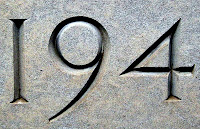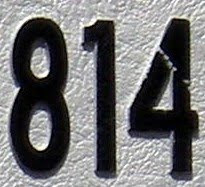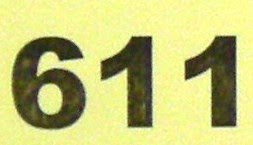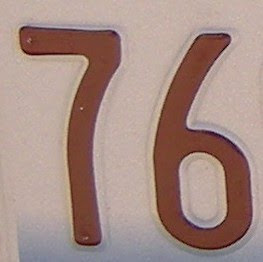## Monday, November 30, 2009

### 57

57 = 3 x 19.

57 is 111 in base 7.

57 is the sum of the 7th through 12th numbers: 7 + 8 + 9 + 10 + 11 + 12 = 57.

As a semiprime (a product of two primes), 57 is a Blum integer because its two prime factors, 3 and 19, are both Gaussian primes."57 Varieties" was the slogan of Pittsburgh's H. J. Heinz Company. In its early days, the company wanted to advertise the great number of choices of canned and bottled foods it offered for sale. Although the company had more than 60 products in 1892, the number 57 was chosen because the number "5" was Henry John Heinz's lucky number and the number "7" was his wife's lucky number.

Source: Wikipedia

## Wednesday, November 25, 2009

### 194

194 = 2 x 97.

194 has a representation as a sum of two squares: 194 = 52 + 132.

194 is the smallest number with five representations as a sum of three squares: 194 = 12 + 72 + 122 = 32 + 42 + 132 = 32 + 82 + 112 = 52 + 52 + 122 = 72 + 82 + 92.

194 is a number n such that n4 + 1 is prime: 1,416,468,497 is prime.194 Prokne is a large, dark, main-belt asteroid discovered in 1879.

Source:

## Tuesday, November 24, 2009

### 271

271 is a prime number.

269 and 271 form a twin prime pair.

271 is a number that cannot be written as a sum of three squares.

271 is the sum of eleven consecutive primes: 271 = 7 + 11 + 13 + 17 + 19 + 23 + 29 + 31 + 37 + 41 + 43.

271 is a Google number. The nth Google number is the first n-digit prime found in the decimal expansion of e. This sequence of numbers was originally featured in an unusual job ad placed by Google. The first few numbers in the sequence are
• 2,
• 71,
• 271,
• 4523,
• 74713,
• 904523, . . .

• ## Monday, November 23, 2009

### 929

929 is a prime number.

929 is the smallest palindromic prime whose cube can be expressed as the sum of three odd cubes: 9293 = 693 + 4473 + 8933.

929 has a representation as a sum of two squares: 929 = 202 + 232.

929 is the hypotenuse of a primitive Pythagorean triple: 9292 = 1292 + 9202.

The Mazda 929 automobile was introduced in 1973.

## Friday, November 20, 2009

### 799

799 = 17 x 47.

799 is the smallest number with a composite sum of digits (7 + 9 + 9 = 25) and a prime sum of cubes of digits (73 + 93 + 93 = 1801).

799 is the smallest number whose sum of digits is 25.

799 is a number that cannot be written as a sum of three squares.

799 is the sum of 11 positive fifth powers: 799 = 35 + 35 + 35 + 25 + 25 + 15 + 15 + 15 + 15 + 15 + 15.

NGC 799 is a spiral galaxy in the constellation Cetus.

## Thursday, November 19, 2009

### 357

357 = 3 x 7 x 17.

357 is the only three-digit number such that 2*357357 - 1 is prime.

357 is the smallest three-digit number with its digits being three distinct odd primes that is not prime, nor do any of the permutations of its digits represent a prime number.

357 is the larger of the only two sphenic numbers (product of three distinct primes) consisting of three distinct prime digits.

357 is a divisor of 502 - 1.

The .357 Magnum is a revolver cartridge.

## Wednesday, November 18, 2009

### 93

93 = 3 x 31.

93 has the unique representation 93 = 22 + 52 + 82 as a sum of three squares.

93 is 1011101 in base 2 and 333 in base 5.

93 is a cake number. The nth cake number is the maximum number of pieces into which a (cylindrical) cake can be cut with n planar cuts. The first 10 cake numbers are 2, 4, 8, 15, 26, 42, 64, 93, 130, 176. There are 39 cake numbers below 10,000.

The chemical element niobium has one stable isotope, which has 93 neutrons.

## Tuesday, November 17, 2009

### 1019

1019 is a prime number.

1019 is the number of twin prime pairs between 10,000 and 100,000.

1019 and 1021 form a twin prime pair.

1019 is a Sophie Germain prime because 2 x 1019 + 1 = 2039, and 2039 is also a prime.The Broadway musical had an initial run of 1019 performances.

Source: Prime Curios!

## Monday, November 16, 2009

### 814

814 = 2 x 11 x 37.

29 circles divide the plane into at most 814 regions.

814 is a value of n such that n(n + 5) is a palindrome: 814 x 819 = 666666.

814 is a member of the sequence generated by starting with 1, then repeatedly reversing the digits and adding 13: 1, 14, 54, 98, . . . , 108, 814, 431, . . .814 is the numerical designation of a naval air squadron of the Royal Navy Fleet Air Arm nicknamed the "Flying Tigers."

## Friday, November 13, 2009

### 989

989 = 23 x 43.

989 is the smallest number so that it and its reverse are divisible by 43.

989 is 33131 in base 4 and 12424 in base 5.

989 is one of the integers generated by the following sieve: Keep first number, drop every second, keep first, drop every third, keep first, drop every fourth, and so on: 0, 1, 3, 5, 9, 11, 17, 21, 29, 33, . . . , 989, . . .Halley's Comet was visible from Earth in the year 989.

## Thursday, November 12, 2009

### 479

479 is a prime number. Moreover, the differences between any two of its digits are also prime.

479 is a number that cannot be written as a sum of three squares.

479 is the largest known prime number that cannot be represented as the sum of fewer than nineteen fourth powers.

Inserting 0 between adjacent digits of 479 produces a prime: 40709.479 is the telephone area code serving northwestern Arkansas.

Source: Prime Curios!

## Wednesday, November 11, 2009

### 506

506 = 2 x 11 x 23.

506 is a pronic number because it is the product of two consecutive integers: 22 x 23 = 506.

506 is the sum of the first eleven squares: 12 + 22 + . . . + 102 + 112 = 506 (which makes it a square pyramidal number).

506 is a hendecagonal number.The telephone area code 506 covers the Canadian province of New Brunswick.

Source: Number Gossip

## Tuesday, November 10, 2009

### 115

115 = 5 x 23.

115 = 23 x (2 + 3).

115 has a unique representation as a sum of three squares: 32 + 52 + 92 = 115.

115 is the smallest three-digit integer, abc, such that (abc)/(a*b*c) is prime: 115/5 = 23.STS-115 was a space shuttle mission to the International Space Station flown by the space shuttle Atlantis on Sept. 9, 2006.Source: Prime Curios!

## Monday, November 9, 2009

### 611

611 = 13 x 47.

611 is a brilliant number. It is a semiprime (product of two primes) whose prime factors have the same number of decimal digits. These numbers are generally used for cryptographic purposes and for testing the performance of prime factoring programs.

611 is a number n such that n times n + 5 gives the concatenation of a number m and itself: 611 x 616 = 376376.

611 is a number with no zeroes that becomes a prime when any one digit is deleted: 61 and 11 are both primes.For customers of some telephone companies in Canada and in the United States, 611 is the abbreviated dialing telephone number used to report a problem with telephone service, or with a payphone.

## Friday, November 6, 2009

### 76

76 = 22 x 19.

76 has a unique representation as the sum of three squares: 76 = 22 + 62 + 62.

76 is the ninth Lucas number. Lucas numbers are the sequence of integers obtained when each successive element is the sum of the previous two elements, starting with 1 and 3.

76 is the number of integer partitions of 21 into distinct parts. The partitions of an integer are the number of ways of writing that integer as a sum of positive integers without regard to order with the constraint that all integers in a given partition are distinct.Meredith Wilson's song "Seventy-six Trombones" is a highlight of the musical The Music Man.

Source: Wolfram MathWorld